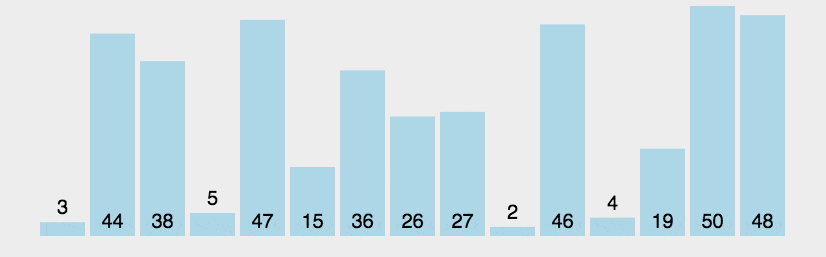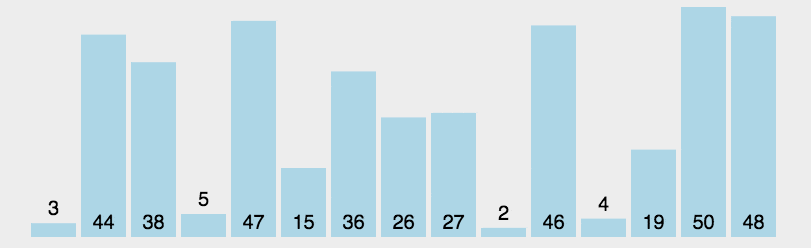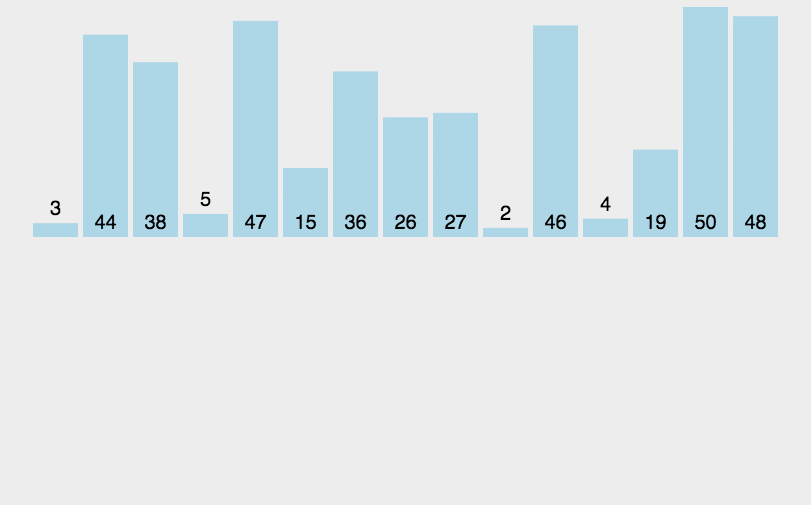# # 经典排序算法

## # 1. 冒泡排序

### # 1.1. 算法步骤• 比较相邻的元素，如果第一个比第二个大（小），就交换他们两个
• 对每一对相邻元素作同样的工作，从开始第一对到结尾的最后一对
• 这步做完后，最后的元素会是最大（小）的数
• 针对所有的元素重复以上的步骤，除了最后一个

### # 1.2. 一轮排序

``````/**
* 先写出一轮的冒泡排序处理，冒泡一样的交换位置到最后一位
*
* 大于就交换位置是从小到大，每次大于后面的值才交换，最后一位是最大值
* 小于就交换位置是从大到小，每次小于后面的值才交换，最后一位是最小值
*
* array.length - 1，不然会超出边界，因为 array[j + 1]
*
* 当然也可以是从j = 1开始，j和j - 1进行比较，这样就不用array.length - 1了
* 大于和小于也需要调换，大于会变成从大到小，小于会变成从小到大
* 当然这种逆向的理解起来比较复杂一点，第二块代码是逆向的写法
*
* @param array
*/
private static void bubbleSort1(int[] array) {
print(array);

for (int j = 0; j < array.length - 1; j++) {
if (array[j] > array[j + 1]) {
int temp = array[j];
array[j] = array[j + 1];
array[j + 1] = temp;
}
}

/*for (int j = 1; j < array.length; j++) {
if (array[j] < array[j - 1]) {
int temp = array[j];
array[j] = array[j - 1];
array[j - 1] = temp;
}
}*/

print(array);
}
``````

``````public static void main(String[] args) {
int[] array = {5, 3, 2, 4, 9, 8, 7, 1, 6};
System.out.println("---------- bubbleSort1 ----------");
bubbleSort1(Arrays.copyOf(array, array.length));
}
``````
``````---------- bubbleSort1 ----------
5 3 2 4 9 8 7 1 6
3 2 4 5 8 7 1 6 9
``````

### # 1.3. 基础版本

``````/**
* 基于一轮的冒泡排序处理，再增加一个外循环
* 将每个位置的数字都进行一轮冒泡交换
* 这样一个最基础的冒泡排序就完成了
*
* 给定一个count记录循环次数
*
* @param array
*/
private static void bubbleSort2(int[] array) {
print(array);

// count记录循环次数
int count = 0;

for (int i = 0; i < array.length; i++) {
for (int j = 0; j < array.length - 1; j++) {
count++;
if (array[j] > array[j + 1]) {
int temp = array[j];
array[j] = array[j + 1];
array[j + 1] = temp;
}
}
}
System.out.println("count: " + count);

print(array);
}
``````

``````public static void main(String[] args) {
int[] array = {5, 3, 2, 4, 9, 8, 7, 1, 6};
System.out.println("---------- bubbleSort2 ----------");
bubbleSort2(Arrays.copyOf(array, array.length));
}
``````
``````---------- bubbleSort2 ----------
5 3 2 4 9 8 7 1 6
count: 72
1 2 3 4 5 6 7 8 9
``````

### # 1.4. 优化版本

``````/**
* 优化版本，内循环减去i就行了
* 每次冒泡后到i位置的数字都已经排序好了
* 所以就没必要重复排
*
* 可以看到count次数少了一半
*
* @param array
*/
private static void bubbleSort3(int[] array) {
print(array);

// count记录循环次数
int count = 0;

for (int i = 0; i < array.length; i++) {
for (int j = 0; j < array.length - i - 1; j++) {
if (array[j] > array[j + 1]) {
int temp = array[j];
array[j] = array[j + 1];
array[j + 1] = temp;
}
count++;
}
}

// 还有一种外循环i反过来循环的，意思差不多，就是逆向的
/*for (int i = array.length - 1; i > 0 ; i--) {
for (int j = 0; j < i; j++) {
count++;
if (array[j] > array[j + 1]) {
int temp = array[j];
array[j] = array[j + 1];
array[j + 1] = temp;
}
}
}*/
System.out.println("count: " + count);

print(array);
}
``````

``````public static void main(String[] args) {
int[] array = {5, 3, 2, 4, 9, 8, 7, 1, 6};
System.out.println("---------- bubbleSort3 ----------");
bubbleSort3(Arrays.copyOf(array, array.length));
}
``````
``````---------- bubbleSort3 ----------
5 3 2 4 9 8 7 1 6
count: 36
1 2 3 4 5 6 7 8 9
``````

### # 1.5. 优化版本v2

``````/**
* 优化版本v2，假定数组某些数已经有序
* 在每轮交换处理可以定一个标记，如果这轮比较都没有交换位置的话
* 说明数据已经有序，可以直接结束
*
* 给定一个count记录循环次数
* 换一个存在某些数有序的数组，可以看到count次数又会变少
*
* @param array
*/
private static void bubbleSort4(int[] array) {
print(array);

// count记录循环次数
int count = 0;

for (int i = 0; i < array.length; i++) {
// 设定一个标记
boolean mark = Boolean.TRUE;

for (int j = 0; j < array.length - i - 1; j++) {
if (array[j] > array[j + 1]) {
int temp = array[j];
array[j] = array[j + 1];
array[j + 1] = temp;
mark = Boolean.FALSE;
}
count++;
}

// 若为true，则表示此次循环没有进行交换
if (mark) {
// 也就是待排序列已经有序，排序已经完成，直接结束
break;
}
}

System.out.println("count: " + count);

print(array);
}
``````

``````public static void main(String[] args) {
// int[] array = {5, 3, 2, 4, 9, 8, 7, 1, 6};
System.out.println("---------- bubbleSort4 ----------");
int[] array4 = {4, 1, 2, 3, 9, 8, 7, 5, 6};
bubbleSort4(Arrays.copyOf(array4, array.length));
}
``````
``````---------- bubbleSort4 ----------
4 1 2 3 9 8 7 5 6
count: 26
1 2 3 4 5 6 7 8 9
``````

### # 1.6. 算法总结

• 描述
• 无序区，有序区
• 从无序区通过交换找到最大（小）元素放到有序区前端
• 稳定性
• 稳定的
• 时间复杂度
• 最好应该是线性阶 O(n)
• 最差每次就进行排序为平方阶 O(n^2)
• 所以平均复杂度为平方阶 O(n^2)
• 空间复杂度
• 没有占用额外空间，所以为常数阶 O(1)

## # 2. 选择排序

### # 2.1. 算法步骤• 首先在未排序序列中找到最小（大）元素，存放到已排序序列的起始位置
• 再从剩余未排序元素中继续寻找最小（大）元素，然后放到已排序序列的下一个位置
• 重复第二步，直到所有元素均排序完毕

### # 2.2. 一轮排序

``````/**
* 先写出一轮的选择排序处理
*
* 可以看到最小的数字交换到第一个了
*
* @param array
*/
private static void selectionSort1(int[] array) {
print(array);

// 记录最小下标位置
int minIndex = 0;

for (int j = 0; j < array.length; j++) {
if (array[j] < array[minIndex]) {
minIndex = j;
}
}

// 将最小下标值进行交换
int temp = array;
array = array[minIndex];
array[minIndex] = temp;

print(array);
}
``````

``````public static void main(String[] args) {
int[] array = {5, 3, 2, 4, 9, 8, 7, 1, 6};
System.out.println("---------- selectionSort1 ----------");
selectionSort1(Arrays.copyOf(array, array.length));
}
``````
``````---------- selectionSort1 ----------
5 3 2 4 9 8 7 1 6
1 3 2 4 9 8 7 5 6
``````

### # 2.3. 基础版本

``````/**
* 基于一轮的选择排序处理，再增加一个外循环
* 将每个位置的数字都进行一轮选择交换
* 这样一个最基础的选择排序就完成了
*
* @param array
*/
private static void selectionSort2(int[] array) {
print(array);

// count记录循环次数
int count = 0;

for (int i = 0; i < array.length; i++) {
// 记录最小下标位置
int minIndex = i;

for (int j = i; j < array.length; j++) {
count++;
if (array[j] < array[minIndex]) {
minIndex = j;
}
}

// 将最小下标值进行交换
int temp = array[i];
array[i] = array[minIndex];
array[minIndex] = temp;
}

System.out.println("count: " + count);

print(array);
}
``````

``````public static void main(String[] args) {
int[] array = {5, 3, 2, 4, 9, 8, 7, 1, 6};
System.out.println("---------- selectionSort2 ----------");
selectionSort2(Arrays.copyOf(array, array.length));
}
``````
``````---------- selectionSort2 ----------
5 3 2 4 9 8 7 1 6
count: 45
1 2 3 4 5 6 7 8 9
``````

### # 2.4. 优化版本

``````/**
* 优化版本，边界处理优化
*
* 应该从 j = i + 1 开始，i为0时，如果j = i
* 那就是array和array比较了一次
*
* 外循环也应该i < array.length - 1，也是一样
* 不进行-1的话结尾array和array多比较了一次
*
* @param array
*/
private static void selectionSort3(int[] array) {
print(array);

// count记录循环次数
int count = 0;

for (int i = 0; i < array.length - 1; i++) {
// 记录最小下标位置
int minIndex = i;

for (int j = i + 1; j < array.length; j++) {
if (array[j] < array[minIndex]) {
minIndex = j;
}
count++;
}

// 将最小下标值进行交换
int temp = array[i];
array[i] = array[minIndex];
array[minIndex] = temp;
}

System.out.println("count: " + count);

print(array);
}
``````

``````public static void main(String[] args) {
int[] array = {5, 3, 2, 4, 9, 8, 7, 1, 6};
System.out.println("---------- selectionSort3 ----------");
selectionSort3(Arrays.copyOf(array, array.length));
}
``````
``````---------- selectionSort3 ----------
5 3 2 4 9 8 7 1 6
count: 36
1 2 3 4 5 6 7 8 9
``````

### # 2.5. 优化版本v2

``````/**
* 优化版本v2，交换可以优化，不是每次都需要交换
*
* 如果下标是一样的，说明当前数就是最小的
* 没必要自己和自己交换了
*
* @param array
*/
private static void selectionSort4(int[] array) {
print(array);

// count记录循环次数
int count = 0;

for (int i = 0; i < array.length - 1; i++) {
// 记录最小下标位置
int minIndex = i;

for (int j = i + 1; j < array.length; j++) {
if (array[j] < array[minIndex]) {
minIndex = j;
}
count++;
}

// 最小下标位置和i不相等说明找到新的最小数才进行交换
if (minIndex != i) {
int temp = array[i];
array[i] = array[minIndex];
array[minIndex] = temp;
}
}

System.out.println("count: " + count);

print(array);
}
``````

``````public static void main(String[] args) {
int[] array = {5, 3, 2, 4, 9, 8, 7, 1, 6};
System.out.println("---------- selectionSort4 ----------");
selectionSort4(Arrays.copyOf(array, array.length));
}
``````
``````---------- selectionSort4 ----------
5 3 2 4 9 8 7 1 6
count: 36
1 2 3 4 5 6 7 8 9
``````

### # 2.7. 算法总结

• 描述
• 无序区，有序区
• 从无序区找到最大（小）元素跟到有序区的后面，对数组比较多，交换少
• 稳定性
• 数组是不稳定的排序方法
• 比如序列 [5， 5， 3] 第一次就将第一个  与  交换，导致第一个 5 挪动到第二个 5 后面
• 链表是稳定的吗？
• 时间复杂度
• 最好是平方阶 O(n^2)
• 最差也是为平方阶 O(n^2)
• 所以平均复杂度为平方阶 O(n^2)
• 空间复杂度
• 没有占用额外空间，所以为常数阶 O(1)

## # 3. 插入排序

• 扑克牌排序，玩过扑克牌的人都能理解
• 队伍排队，从前往后、按高矮顺序排序

### # 3.1. 算法步骤• 将第一待排序序列第一个元素看做一个有序序列，把第二个元素到最后一个元素当成是未排序序列
• 从头到尾依次扫描未排序序列，将扫描到的每个元素插入有序序列的适当位置
• 如果待插入的元素与有序序列中的某个元素相等，则将待插入元素插入到相等元素的后面

### # 3.2. 一轮排序

``````/**
* 先写出一轮的插入插入处理
*
* 设定是从第2个数往前插入
*
* @param array
*/
private static void insertionSort1(int[] array) {
print(array);

int current = array;

// 从第2个开始比较，没有小于的数据后结束循环进行插入
int j = 2;
while (j > 0 && (current < array[j - 1])) {
array[j] = array[j - 1];
j--;
}

// j不等于2，说明找到了比其小的数，才进行插入
if (j != 2) {
array[j] = current;
}

// 使用交换的方式
/*for (int j = 2; j > 0; j--) {
if (array[j] < array[j - 1]) {
int temp = array[j];
array[j] = array[j - 1];
array[j - 1] = temp;
}
}*/

print(array);
}
``````

``````public static void main(String[] args) {
int[] array = {5, 3, 2, 4, 9, 8, 7, 1, 6};
System.out.println("---------- insertionSort1 ----------");
insertionSort1(Arrays.copyOf(array, array.length));
}
``````
``````---------- insertionSort1 ----------
5 3 2 4 9 8 7 1 6
2 5 3 4 9 8 7 1 6
``````

### # 3.2. 基础版本

``````/**
* 基于一轮的插入排序处理，再增加一个外循环
*
* 这样一个最基础的插入排序就完成了
*
* @param array
*/
private static void insertionSort2(int[] array) {
print(array);

for (int i = 0; i < array.length; i++) {
int current = array[i];

// 从后往前的开始比较，没有小于的数据后结束循环进行插入
int j = i;
while (j > 0 && (current < array[j - 1])) {
array[j] = array[j - 1];
j--;
}

// j和i不一致说明找到了比其小的数，才进行插入
if (j != i) {
array[j] = current;
}
}

// 使用交换的方式
/*for (int i = 0; i < array.length; i++) {
for (int j = i + 1; j > 0; j--) {
if (array[j] < array[j - 1]) {
int temp = array[j];
array[j] = array[j - 1];
array[j - 1] = temp;
}
}
}*/

print(array);
}
``````

``````public static void main(String[] args) {
int[] array = {5, 3, 2, 4, 9, 8, 7, 1, 6};
System.out.println("---------- insertionSort2 ----------");
insertionSort2(Arrays.copyOf(array, array.length));
}
``````
``````---------- insertionSort2 ----------
5 3 2 4 9 8 7 1 6
1 2 3 4 5 6 7 8 9
``````

### # 3.3. 优化版本

``````/**
* 优化版本，边界处理
* i可以直接从1开始，0的只有一个元素无需处理
*
* 交换的方式的话，优化i < array.length - 1
*
* @param array
*/
private static void insertionSort3(int[] array) {
print(array);

// 从下标为1的元素开始选择合适的位置插入，因为下标为0的只有一个元素无需处理
for (int i = 1; i < array.length; i++) {
int current = array[i];

// 从后往前的开始比较，没有小于的数据后结束循环进行插入
int j = i;
while (j > 0 && (current < array[j - 1])) {
array[j] = array[j - 1];
j--;
}

// j和i不一致说明找到了比其小的数，才进行插入
if (j != i) {
array[j] = current;
}
}

// 使用交换的方式
/*for (int i = 0; i < array.length - 1; i++) {
for (int j = i + 1; j > 0; j--) {
if (array[j] < array[j - 1]) {
int temp = array[j];
array[j] = array[j - 1];
array[j - 1] = temp;
}
}
}*/

print(array);
}
``````

``````public static void main(String[] args) {
int[] array = {5, 3, 2, 4, 9, 8, 7, 1, 6};
System.out.println("---------- insertionSort3 ----------");
insertionSort3(Arrays.copyOf(array, array.length));
}
``````
``````---------- insertionSort3 ----------
5 3 2 4 9 8 7 1 6
1 2 3 4 5 6 7 8 9
``````

• 拆半插入(二分法拆入)

### # 3.5. 算法总结

• 描述
• 无序区，有序区
• 把无序区的元素插入到有序区合适的位置，对数组比较少，交换多
• 稳定性
• 稳定的
• 时间复杂度
• 最好是线性阶 O(n)
• 最差为平方阶 O(n^2)
• 所以平均复杂度为平方阶 O(n^2)
• 空间复杂度
• 没有占用额外空间，所以为常数阶 O(1)